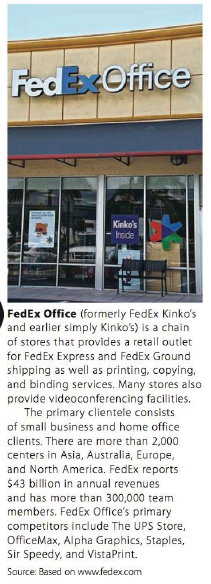Chapter 5.II, Problem 22RE### Contemporary Mathematics for Busin...

8th Edition
Robert Brechner + 1 other
ISBN: 9781305585447

#### Solutions

Chapter
Section### Contemporary Mathematics for Busin...

8th Edition
Robert Brechner + 1 other
ISBN: 9781305585447
Textbook Problem

# Use ratio and proportion to solve the following business situations.A local FedEx Office store has a press that can print 5,800 brochures per hour. How many can be printed during a 3 1 4 -hour run?To determine

To calculate: The number of brochures that can be printed in 314 hours by a local FedEx office store, if 5,800 brochures can be printed in 1 hour.

Explanation

Given Information:

The FedEx office store can print 5,800 brochures in 1 hour.

Formula used:

Cross multiplication method:

To solve the proportional problem by using cross multiplication, follow the steps as mentioned below.

Step 1: Represent the unknowns by a letter.

Step 2: Set the proportion with one ratio, expressed as a fraction on both side of the equal sign.

Step 3: Multiply numerator of the left side ratio with the denominatior of right side ratio and place the product on one side of the equal sign.

Step 4: Multiply denominatior of the left side ratio with the nominatior of right side ratio and place the product on other side of the equal sign.

The step 3 and 4 together is called as cross multiplication.

Step5: Solve for the unknown value.

Calculation:

Consider the number of brochures printed in 1 hour is 5,800.

Suppose that X be the number of brochures can be printed in 314 hours.

The proportion is 5,800 brochures is to 1 hour equals X brochures is to 314 hours,

58001=X

Apply cross multiplication, this gives,

(5800)314=(1)X(5800)

### Still sussing out bartleby?

Check out a sample textbook solution.

See a sample solution

#### The Solution to Your Study Problems

Bartleby provides explanations to thousands of textbook problems written by our experts, many with advanced degrees!

Get Started

#### Find more solutions based on key concepts